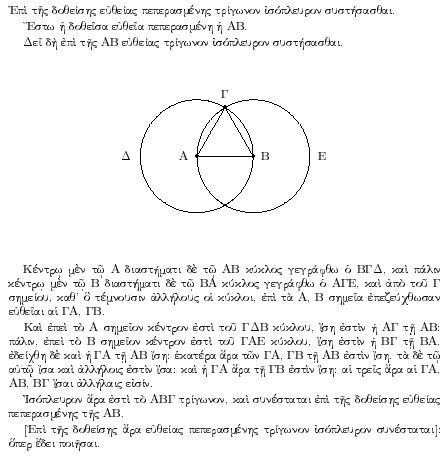# Mathematics as art and science

Mathematics is a living science, where problems and theory come from the discipline itself. As such, pure mathematics can be considered both as an art and a science. Mathematics is actually much more than just a tool and methodology!A proof from Euclid's elements, which says that given a line segment, one can find an equilateral triangle with the line segment as one of the sides. The proof is by construction: an equilateral triangle ABΓ can be obtained by drawing the circles Δ and E with centres at the points A and B, and then taking one of the intersection points of the circles as the third corner in the triangle.
Photo:
Wikipedia

## Main content

Mathematics is one of the oldest sciences. It flourished in ancient Greece, where we find the first serious attempt at axiomatisation and logical proof, particularly in Euclidean geometry. In the Middle Ages, mathematics survived in the first universities and in the Arab world. In the 1600s, mathematical analysis developed through the works of René Descartes, Isaac Newton and Gottfried Leibniz. At this time, research in mathematics was strongly associated with problems from the physical world, but in the subsequent years, mathematics became more specialised and professionalised and we obtained a clearer distinction between pure and applied mathematics.

Mathematical thinking and language have influenced our culture throughout all time. The numerals were among the first words to appear in human history. Gradually, the human brain has produced more and more fascinating problems both out of pure curiosity and also inspired by other sciences. Such problems have haunted mathematicians for hundreds of years.